This site uses cookies to deliver our services, improve performance, for analytics, and (if not signed in) for advertising. By using LibraryThing you acknowledge that you have read and understand our Terms of Service and Privacy Policy. Your use of the site and services is subject to these policies and terms.

Random books from Eduardo.del.Olmo's library

Lie algebras in particle physics by Howard Georgi

Calculus by Michael Spivak

Differential Geometry and Lie Groups for Physicists by Marián Fecko

Quantum Chromodynamics by Walter Greiner

Theory of Algebraic Invariants (Cambridge Mathematical Library) by David Hilbert

Member: Eduardo.del.Olmo

CollectionsFiction (750), Algebra, General (755), Analysis, Calculus (725), Analysis, Complex (622), Analysis, Functional (732), Analysis, Numerical (573), Arithmetic (1), Astronomy and Astrophysics (526), Biochemistry (1), Biology (4), Chemistry (729), Combinatorics (2), Computation (654), Cosmology (570), Difference and Differential Equations (680), Differential Geometry (674), Discrete Mathematics (636), Electricitrodynamics (5), Electromagnetism (662), Engineering (644), Field Theory (physics) (659), Fluids (51), General Textbook (578), Geometry (717), Grammar (3), Group Theory (687), History of Mathematics (707), Logic (3), Manifolds (637), Mathematical Physics (3), Mathematics (936), Mechanics of Continuum and Elasticity (5), Mechanics, Classical (556), Mechanics, Engineering (583), Number Theory (632), Optics (695), Particle Physics (690), Physics (959), Plasma Physics (1), Popular (568), Probability and Statistics (592), Quantum Computation (1), Quantum Field Theory (672), Quantum Physics (784), Relativity (762), Set Theory (1), Solid State (691), Statistical Physics (4), Spectroscopy (1), String Theory (561), Symmetries (1), Thermodynamics (11), Topology (650), Trigonometry (1), Waves (655), Statistics (4), Your library (1,243), To read (741), Favorites (448), Recommended (719), All collections (1,267)

ReviewsNone

TagsPhysics (417), Mathematics (400), Quantum Physics (171), Algebra (139), Analysis (91), Mathematical Physics (81), Geometry (80), Particle Physics (49), Schaum's Outline (45), Nuclear Physics (40) — see all tags

MediaBook (1,267), Paper Book (1,259), Ebook (7)

Cloudstag cloud, author cloud, tag mirror

GroupsNone

Favorite authorsNot set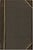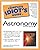Eduardo.del.Olmo rated, added: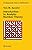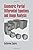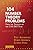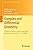Comment Wall|All comments